Count Number Of Duplicates Elements Present In Array

Count Number Of Duplicates Elements Present In Array

You need to count Number of duplicates elements present in an array that occurs two or more times.

For Example:

Input: arr = 1,2,3,1,1,2,4,5,2,3
Output :      3  (1 present 3 times, 2 present 3 times , 3 present 2 times )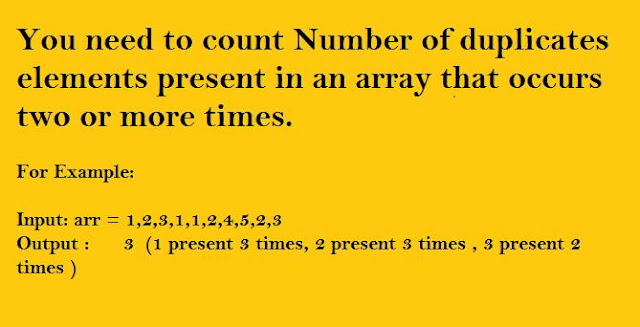Count-Duplicates-In-Array

#include<iostream>
#include<stdio.h>
using namespace std;
int main()
{
int arr[] = { 1,2,3,1,1,4,5,6,4,4,5,2,2,12};
int size1 = sizeof(arr)/sizeof(arr);
int count1 = 0,flag=0, temp = 0;;
int n = 0;

/*first sort array elements so that all duplicates will be next to each other*/

for (int i = 0; i<size1; i++)
{
for (int j = 0; j<size1 - i - 1; j++)
{
if (arr[j] > arr[j + 1])
{
temp = arr[j];
arr[j] = arr[j + 1];
arr[j + 1] = temp;
}
}
}

/*Print sorted array*/

for (int i = 0; i<size1; i++)
cout << arr[i] << "  ";
int i = 0;
while(i<size1)
{
flag = 0;
for (int j = i + 1; j<=size1; j++)
{
if (arr[i] == arr[j])
{
n++;
flag++;
if (flag ==1)
count1++;
continue;
/*if elements present the continue to next iteration inside nested loop*/
}
else
{
n++;
break;
}
}
i = n;
}
cout << "\n\n count value:-  " << count1;
getchar();

}

Delete All Node From A Link List Greater Than A Given Value(X)

Delete All Node From Link List Greater Than A Given ValueLinked-List

Program:

void DeleteNode(node** head, int x)
{

node *ptr = *head;
node *prev,*temp,*temp1;
{
printf("\n\nlist is empty");
}
else if(ptr->data >x)
{
temp = ptr;
ptr=ptr->next;
free(temp);
}
else
{
temp = ptr;
prev = ptr;
printf("\n data :  %d",temp->data);
while(temp!=NULL)
{

if (temp->data >x)
{
temp1 = temp;
temp=temp->next;
prev->next = temp;
free(temp1);
temp =prev;

}
else
{
prev=temp;
temp=temp->next;
}
}

}
}

Other Blogs: Follow here

A Program to check if strings are rotations of each other or not

A Program to check if strings are rotations of each other or not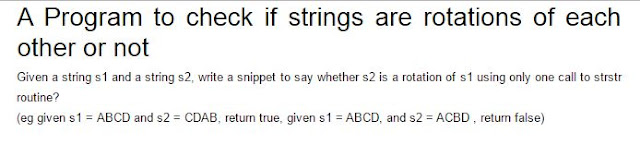String-Rotations #include #include #include using namespace std; void CompareString(string, string, int); int ComputeLength(string str); int main() { string str = ""; string str1 = ""; int len = 0, len1 = 0; cout << "\nenter string "; cin >> str; cout << "\nenter string 2 to compare:-  "; cin >> str1; len = ComputeStringLength(str); len1 = ComputeStringLength(str1); if (len == len1) CompareString(str, str1, len); else cout << "rotation not possible"; getchar(); return 0; } int ComputeStringLength(string str) { int len = 0; for (int i = 0; str[i] != '\0'; i++) { len++; } return len; } void  CompareString(string str, string str1, int n) { int index = 0, flag = 0, curr_index = 0, count1 = 0, flagj = 0; for (int i = 0; i=0) { int k = 0; for (int i = n - 1; i>n - curr_index - 1; i--) { if (str[i] == str1[k]) { temp++; k++; } } } if (temp == n) { cout << "\n\nstring is same after rotation"; } else { cout << "\n\nstring is not same after rotation"; } } else { cout << "\n\nstring is same after rotation"; } } Other Blogs: Follow here

Interview Question #1

What happens if you Call a free on a pointer twice?

Deallocating a memory area with free does not make the contents of the pointer NULL.

Suppose that you have int *a = malloc (sizeof (int)) and a has 0xdeadbeef and you execute free (a) then after execution a still contains 0xdeadbeef but after the free call this memory address is no more reserved for you.

Something like you have rented a flat with malloc used for some time, returned the flat by free then you might have a duplicate key for the flat, but it is not reserved for you.

Doing a free on an already freed memory will result in double free memory corruption.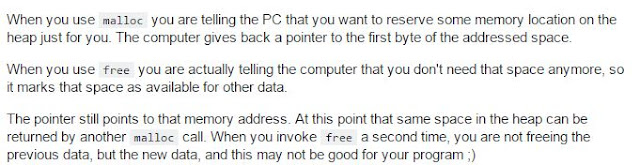Callling-free-twice-on-pointer

Answer Original Source: StackOverFlow

Other Articles:

Article Sources For Learning

Other Blogs: Follow here

Calculate Sum Of All Numbers Present In String

Calculate sum of all numbers present in a stringsum-of-all-digits-present-in-a-string

#include <iostream>
#include<stdio.h>
#include<string.h>
using namespace std;

int SumOfDigits(string str, int n);
int SumOfoverallNumberPresent_In_Sequence(string str, int n);

int main()
{
char str;

printf("Please enter the string:");
gets(str);
int length = strlen(str);
cout<<"\n\nsum of all digits present in string is :-  "<<SumOfDigits(str, length)<<endl;
cout<<"\n\nSum of different numbers present in string:-  "<<SumOfoverallNumberPresent_In_Sequence(str, length)<<endl;
return 0;
}

int SumOfDigits(string str, int n)
{
int i,sum=0,num=0;
for(i = 0;i<=n;i++)
{
if(str[i] >=48 && str[i] <= 57)
{
num = str[i]-48;
sum = sum  + num;
}

}
return sum;
}

int SumOfoverallNumberPresent_In_Sequence(string str, int n)
{
int i,sum=0,digitSum=0,Flag=0,num=0;
for(i = 0;i<=n;i++)
{
if(str[i] >=48 && str[i] <= 57)
{
num = str[i]-48;
sum = sum*10  + num;
}
else
{
digitSum = digitSum+sum;
sum=0;
}

}
return digitSum;
}

Other Articles:

Article Sources For Learning

Other Blogs: Follow here

Kth smallest element in a row-wise and column-wise sorted 2D array

Kth smallest element in a row-wise and column-wise sorted 2D arrayKth-smallest-element

#include<iostream>
#include<stdio.h>
#include<climits>
using namespace std;

typedef struct HeapNode
{
int value;
int row;
int col;
}node;

void minHeapify(node arr[], int n ,int heap_size);
void swap_elements(node *x, node *y);
void  HeapSort(node arr[], int n);
int FindKthElement(int arr,int n,int k);

void swap_elements(node *x, node *y)
{
node z = *x;
*x= *y;
*y=z;
}

void minHeapify(node arr[], int n ,int heap_size)
{
int left = 2*n+1;
int right = 2*n+2;
int small =n;
if(left<heap_size && arr[left].value < arr[n].value)
small =left;
if(right<heap_size && arr[right].value < arr[small].value)
small =right;
if(small !=n)
{
swap_elements(&arr[small], &arr[n]);
minHeapify(arr,small,  heap_size);
}

}

void  HeapSort(node arr[], int n)
{
int i= (n-1)/2;
while(i>=0)
{
minHeapify(arr, i, n);
i--;
}
}

int FindKthElement(int  arr,int n,int k)
{
int next_value =0;
if(k<=0 || k>n*n)
return INT_MAX;

node temp_Arr[n];
/*creating heap for first row elements*/

for (int i = 0; i < n; i++)
temp_Arr[i] = {arr[i], 0, i};
HeapSort(temp_Arr, n);

node Next_value_array;

for(int i=0;i<k;i++)
{
Next_value_array = temp_Arr;

if(Next_value_array.row<(n-1))
{
next_value = arr[Next_value_array.row + 1][Next_value_array.col];
}
else
{
next_value = INT_MAX;
}
//int next_value = (Next_value_array.row<(n-1)) ? arr[Next_value_array.row + 1][Next_value_array.col]:INT_MAX;
temp_Arr =  {next_value, Next_value_array.row+1, Next_value_array.col };
minHeapify(temp_Arr,0, n);
}
return Next_value_array.value;
}

int main()
{
int arr = { {10, 20, 30, 40},
{15, 25, 35, 45},
{25, 29, 37, 48},
{32, 33, 39, 50},
};
cout << "7th smallest element is " << FindKthElement(arr, 4, 7);
return 0;
}

Other Articles:

Compare two strings represented as linked lists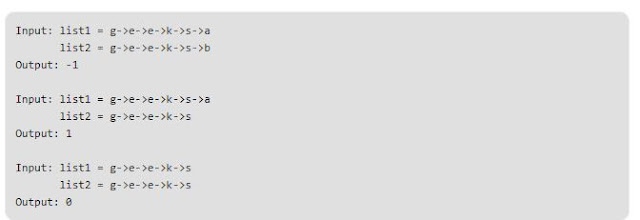Compare Strings

#include<iostream>
#include<stdio.h>
using namespace std;

typedef struct Node
{
char data;
struct Node *next;
}node;

node *CreateList(node* head, char d);
int comparelistString(node *list1, node *list2);

int main()
{
node *list1  = NULL;
node *list2 =  NULL;
list1 = CreateList(list1,'g');
CreateList(list1,'e');
CreateList(list1,'e');
CreateList(list1,'k');
CreateList(list1,'s');
CreateList(list1,'d');

list2 = CreateList(list2,'g');
CreateList(list2,'e');
CreateList(list2,'e');
CreateList(list2,'k');
CreateList(list2,'s');
CreateList(list2,'c');

cout<<"Elements in List 1:-"<<endl;
display(list1);
cout<<"\n\nElements in List 2:-"<<endl;
display(list2);
cout<<endl;

cout<<"\n\ncomparison Result of String is ::  "<<comparelistString(list1, list2)<<"\n\n";

return 0;
}

{
{
}
}

node *CreateList(node* head, char d)
{
node *ptr;
if(ptr==NULL)
{
ptr = new node;
ptr->data = d;
}
else
{
{
}
head->next = new node;

}
}

int comparelistString(node *list1, node *list2)
{
while(list1 !=NULL && list2 !=NULL &&(list1->data == list2->data))
{
list1 = list1->next;
list2 = list2->next;
}
if(list1!=NULL && list2!=NULL)
return((list1->data > list2->data)?1: -1);

if(list1!=NULL && list2==NULL)
return 1;
if (list1==NULL && list2!=NULL)
return -1;

return 0;
}

Output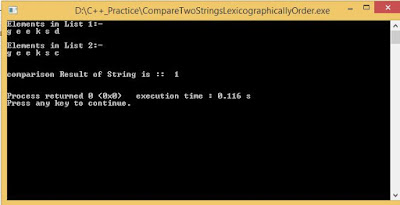Output

Other Articles:

Find merge point of two linked listFind merge point of two linked list

Find merge point of two linked list

#include<iostream>
#include<stdio.h>
using namespace std;

typedef struct Node
{
int data;
struct Node *next;
}node;

int Get_insection_point(int , node*, node*);
int Get_insection_node(node*, node*);
void display(node* ptr);

int main()
{
node* newNode;
( node*) malloc(sizeof( node));

( node*) malloc(sizeof( node));

newNode = ( node*) malloc (sizeof( node));
newNode->data = 6;

newNode = ( node*) malloc (sizeof( node));
newNode->data = 9;

newNode = ( node*) malloc (sizeof( node));
newNode->data = 15;

newNode = ( node*) malloc (sizeof( node));
newNode->data = 30;

cout<<"Elements in List 1 "<<endl<<endl;
cout<<endl;
cout<<"Elements in List 2 "<<endl<<endl;

return 0;
}

void display(node* ptr)
{
while(ptr!=NULL)
{
cout<<ptr->data<< " ";
ptr=ptr->next;
}
cout<<endl<<endl;
}

node* create_list(node* head, int item)
{
node* ptr = head;
if(ptr ==NULL)
{
ptr = (node*)malloc(sizeof(node));
ptr->data = item;
ptr->next = NULL;
}
else
{
while(ptr->next!=NULL)
{
ptr= ptr->next;
}
ptr->next = (node*)malloc(sizeof(node));
ptr= ptr->next;
ptr->data = item;
ptr->next = NULL;
}
return ptr;
}

{
int count1=0;
{
count1++;
}
return count1;
}

{
node * node1Ptr = head1;
node* node2Ptr = head2;
int d=0;
int countC1 = find_count(node1Ptr);
cout<<"no of elements in List 1 :-"<<countC1<<endl;
int countC2 = find_count(node2Ptr);
cout<<"no of elements in List 2 :-"<<countC2<<endl;
if(countC1>countC2)
{
d = countC1-countC2;
return Get_insection_point(d,node1Ptr,node2Ptr );
}
else
{
d= countC2-countC1;
return Get_insection_point(d,node2Ptr,node1Ptr );
}
}

int Get_insection_point(int d , node* head1, node* head2)
{
node * node1Ptr = head1;
node* node2Ptr = head2;

for(int i=0;i<d;i++)
{
if(node1Ptr==NULL)
{
return -1;
}
node1Ptr = node1Ptr->next;
}

while(node1Ptr!=NULL && node2Ptr!=NULL)
{

if(node1Ptr->data == node2Ptr->data)
return node1Ptr->data;
node1Ptr = node1Ptr->next;
node2Ptr = node2Ptr->next;
}
return -1;
}